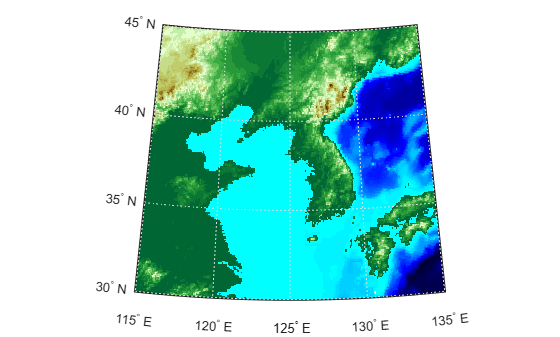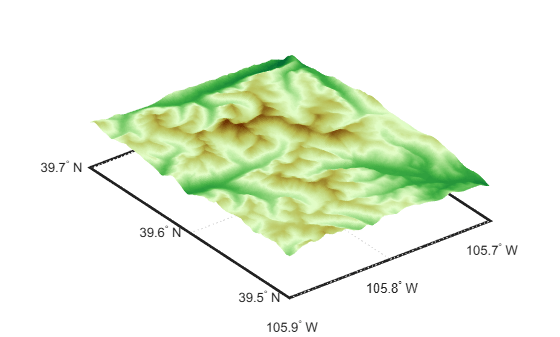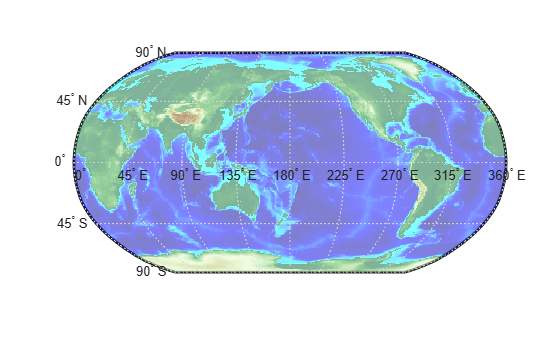# meshm

Project regular data grid on `axesm`-based map

## Syntax

``meshm(Z,R)``
``meshm(Z,R,gratsize)``
``meshm(Z,R,gratsize,h)``
``meshm(___,Name,Value)``
``s = meshm(___)``

## Description

example

````meshm(Z,R)` displays a surface on the current `axesm`-based map by warping the regular data grid specified by `Z`, with spatial reference `R`, to a projected graticule mesh. The color of the surface varies according to the values in `Z`. This syntax displays the surface in a horizontal plane with all height values set to 0.```
````meshm(Z,R,gratsize)` specifies the size of the graticule mesh as `gratsize`.```

example

````meshm(Z,R,gratsize,h)` displays the surface using the heights specified by `h`.```

example

````meshm(___,Name,Value)` specifies surface properties using one or more name-value pair arguments. For example, `"FaceAlpha",0.5` creates a semitransparent plot.```
````s = meshm(___)` returns the primitive surface object. Use `s` to modify the plot after creation. For a list of properties, see Surface Properties.```

## Examples

collapse all

Load elevation data and a geographic cells reference object for the Korean peninsula. Then, display the data on a world map using a colormap appropriate for elevation data.

```load korea5c worldmap(korea5c,korea5cR) meshm(korea5c,korea5cR) demcmap(korea5c)```Read elevation data and a geographic postings reference object for a region around South Boulder Peak in Colorado. Crop the data to cover a smaller region.

```[Z,R] = readgeoraster("n39_w106_3arc_v2.dt1","OutputType","double"); [Z,R] = geocrop(Z,R,[39.5 39.7],[-105.9 -105.7]);```

Display the data as a surface by specifying the graticule size as the size of the raster (`R.RasterSize`) and the height data as the elevation data (`Z`). Apply a colormap appropriate for elevation data.

```usamap(Z,R) meshm(Z,R,R.RasterSize,Z) demcmap(Z)```

View the region in 3-D.

`view(3)`Load world elevation data as an array and a geographic cells reference object. Then, display the data on a world map. Adjust the transparency of the surface plot using the `FaceAlpha` name-value argument.

```load topo60c worldmap(topo60c,topo60cR) meshm(topo60c,topo60cR,"FaceAlpha",0.5)```

Apply a colormap appropriate for elevation data.

`demcmap(topo60c)`## Input Arguments

collapse all

Regular data grid, specified as an m-by-n array.

Spatial reference for `Z`, specified as a `GeographicCellsReference` or `GeographicPostingsReference` object. The `RasterSize` property of `R` must be consistent with `size(Z)`.

Graticule size, specified as one of these options:

• A two-element vector, where the first element indicates the number of parallels and the second element indicates the number of meridians.

• `[]`, which specifies the default graticule size of `[50 100]`. Use this option when you want to use the default graticule size and specify the `h` argument.

Surface height, specified as a scalar or a matrix of size `gratsize`.

• When you specify a scalar, the `meshm` function displays the grid in the horizontal plane with all height values set to `h`.

• When you specify a matrix of size `gratsize`, the `meshm` function displays each vertex of the graticule mesh at the corresponding height in `h`.

When the `MapProjection` property of the `axesm`-based map is `"globe"`, `h` is referenced to the ellipsoid.

Data Types: `double`

### Name-Value Arguments

Specify optional pairs of arguments as `Name1=Value1,...,NameN=ValueN`, where `Name` is the argument name and `Value` is the corresponding value. Name-value arguments must appear after other arguments, but the order of the pairs does not matter.

Example: `meshm(Z,R,LineStyle="-")` displays the graticule mesh using solid lines.

Before R2021a, use commas to separate each name and value, and enclose `Name` in quotes.

Example: `meshm(Z,R,"LineStyle","-")` displays the graticule mesh using solid lines.

Note

Use name-value arguments to specify values for the properties of the `Surface` object created by this function. The properties listed here are only a subset. For a full list, see Surface Properties.

`Surface` objects created by the `meshm` function have these limitations:

• Setting the `XData`, `YData`, and `ZData` properties of the surface object is not supported.

• If you set the `UserData` property of the surface and then change the projection of the `axesm`-based map, MATLAB® does not reproject the surface.

Line style, specified as one of the options listed in this table.

Line StyleDescriptionResulting Line
`"-"`Solid line`"--"`Dashed line`":"`Dotted line`"-."`Dash-dotted line`"none"`No lineNo line

Line width, specified as a positive value in points, where 1 point = 1/72 of an inch. If the line has markers, then the line width also affects the marker edges.

The line width cannot be thinner than the width of a pixel. If you set the line width to a value that is less than the width of a pixel on your system, the line displays as one pixel wide.

## Output Arguments

collapse all

Primitive surface object, returned as a `Surface` object. Use `s` to modify the `Surface` object after creation. For a list of properties, see Surface Properties.

`Surface` objects created by the `meshm` function have these limitations:

• Setting the `XData`, `YData`, and `ZData` properties of the surface object is not supported.

• If you set the `UserData` property of the surface and then change the projection of the `axesm`-based map, MATLAB does not reproject the surface.

## Tips

• The `FaceColor` property of the surface object depends on the size of the graticule mesh. The `meshm` function sets `FaceColor` to `"flat"` when the size of the graticule mesh matches the size of `Z`, and `"texturemap"` otherwise.

• The `meshm` function projects the graticule mesh using the projection specified by the `MapProjection` property of the `axesm`-based map. To improve the resolution of the plot, increase the size of the graticule mesh. Increasing the size of the graticule mesh can increase the amount of time MATLAB requires to display the surface.

## Version History

Introduced before R2006a

expand all